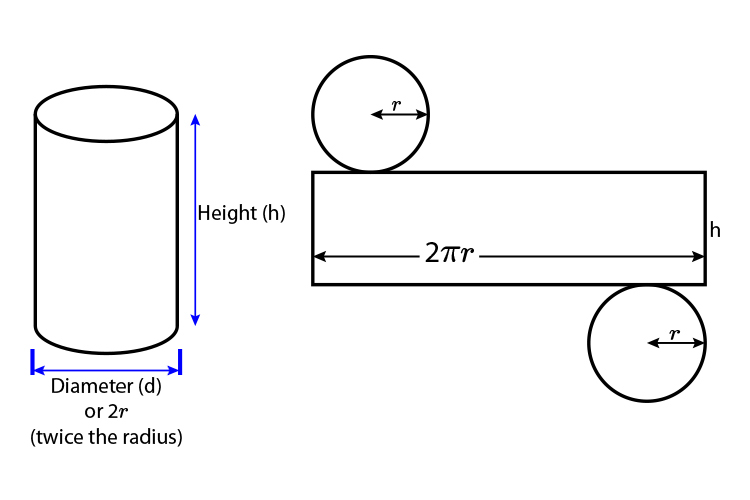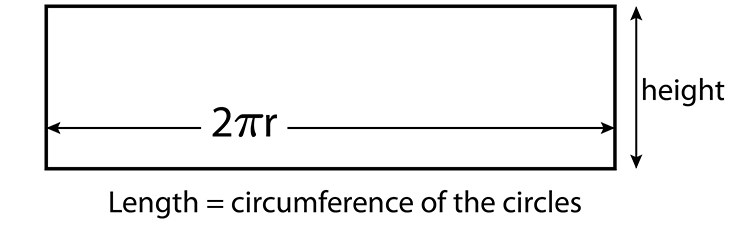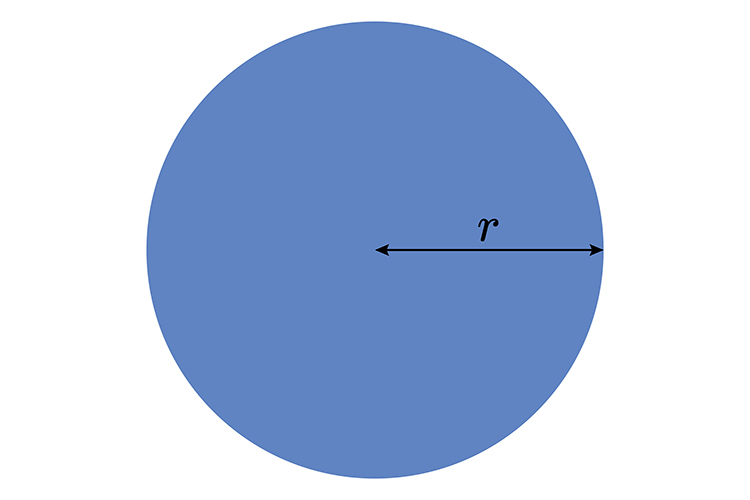# Surface area cylinder

The surface area of any shape can be found by adding together the surface areas of its parts.

## CylinderIf you always imagine pulling apart the cylinder and drawing it out you can clearly see that the areas are one rectangle and two areas of a circle (the top and bottom).

## The rectangleThe area of the rectangle is Height = h   multiplied by Length = 2\pir

Area of rectangle = h\times\2\pir

The circle areas are:Area = \pir^2

And because there are two circles

Area = 2\pir^2

Summary

Area of a cylinder:

h2\pir + 2\pir^2

Example 1

Ted has a cylinder of height 15 cm and diameter 8 cm. He calculates the curved surface area as
2×\pi\times8×15. Explain what he has done wrong.

He used the diameter not the radius (Circumference = 2\pir)
He incorrectly multiplied by 2 when calculating the circumference (Circumference = \pid).